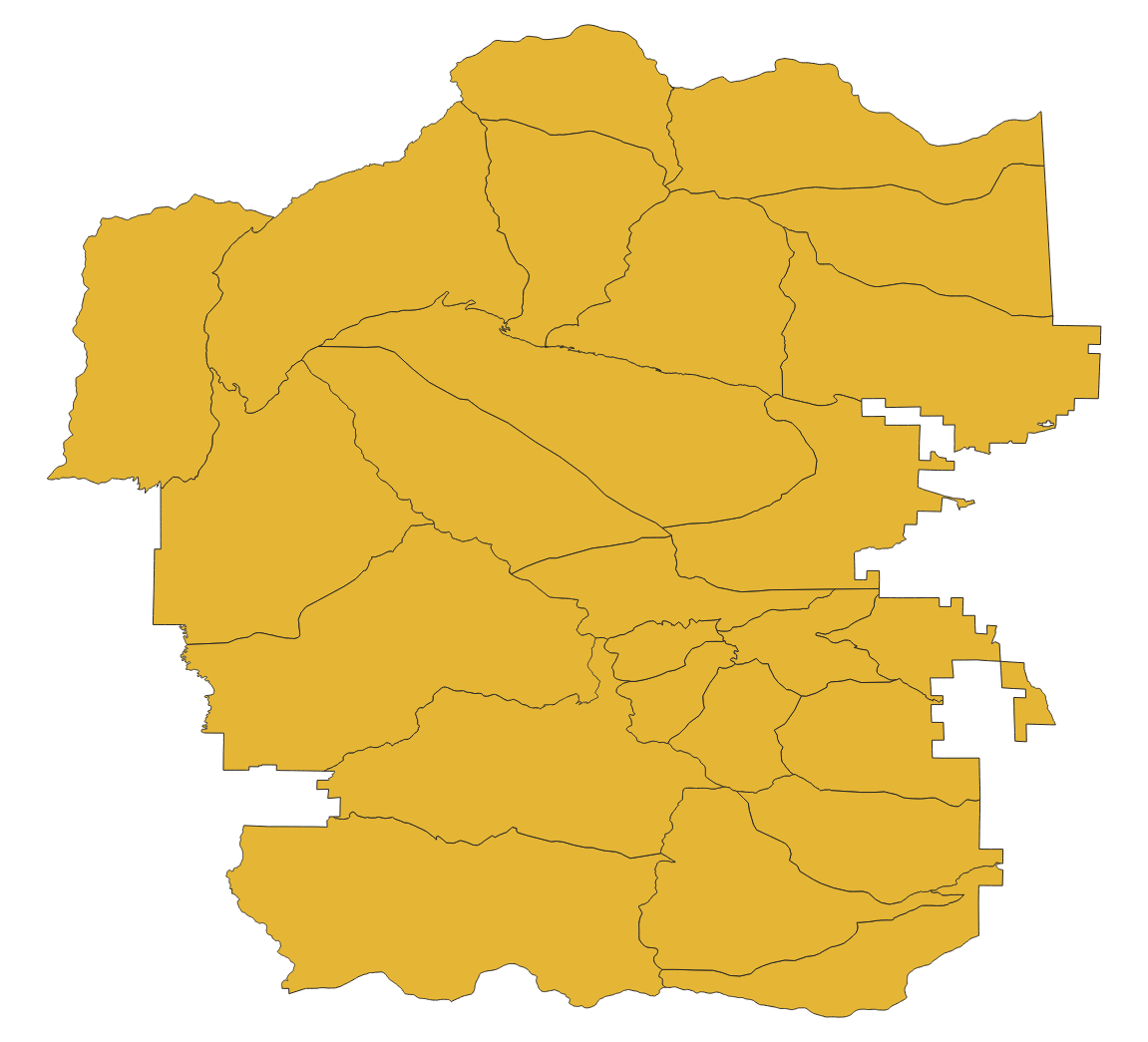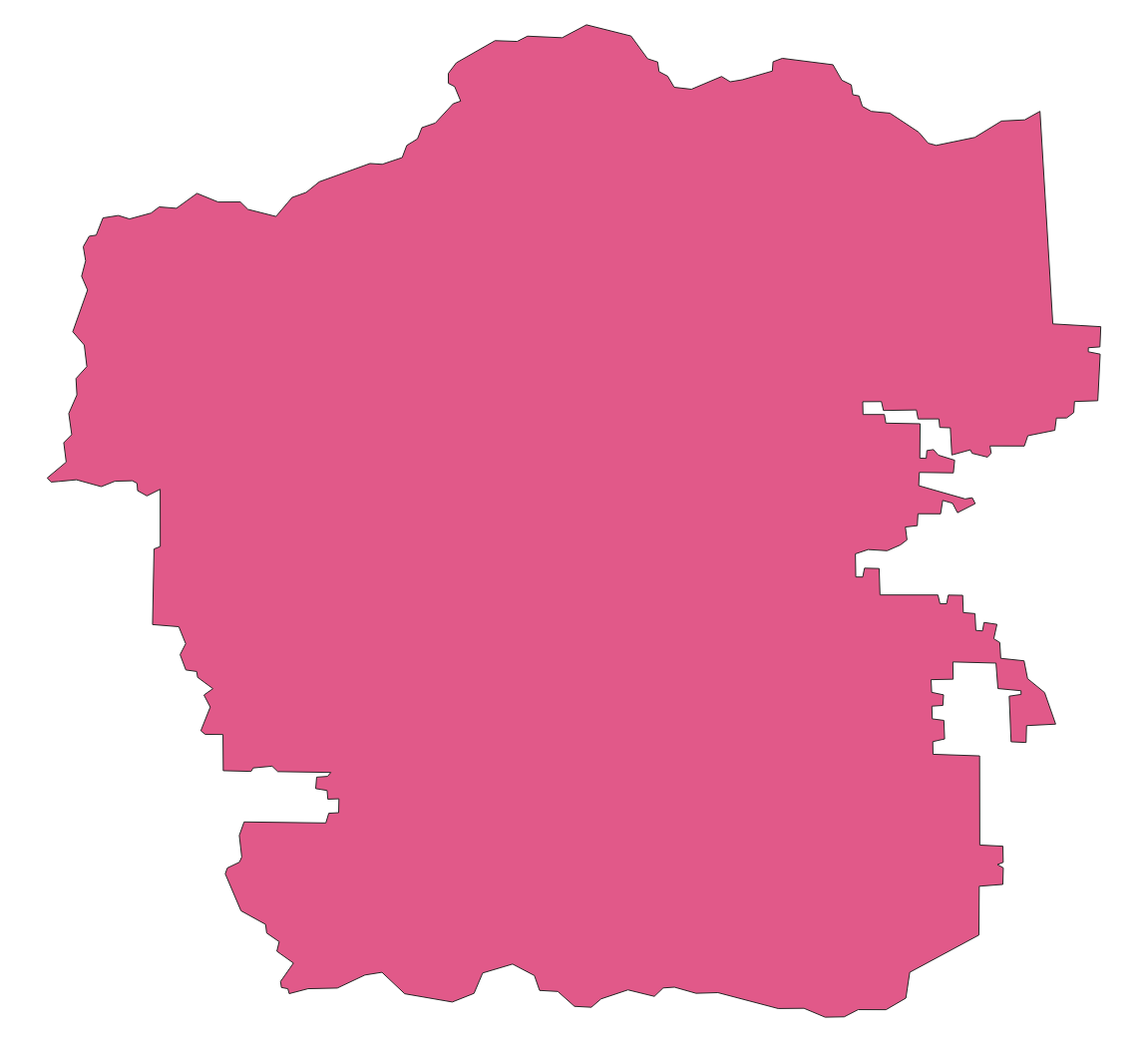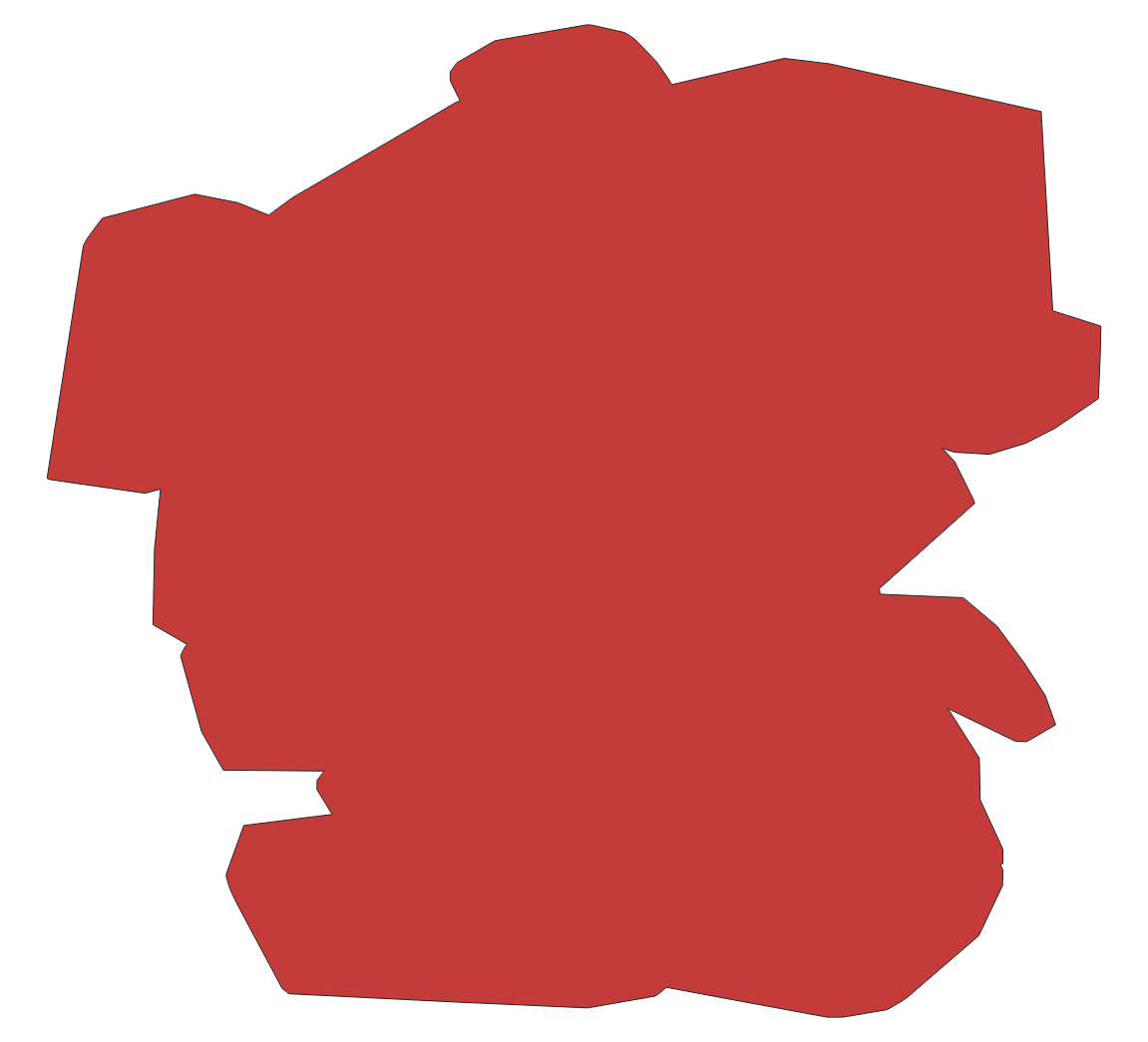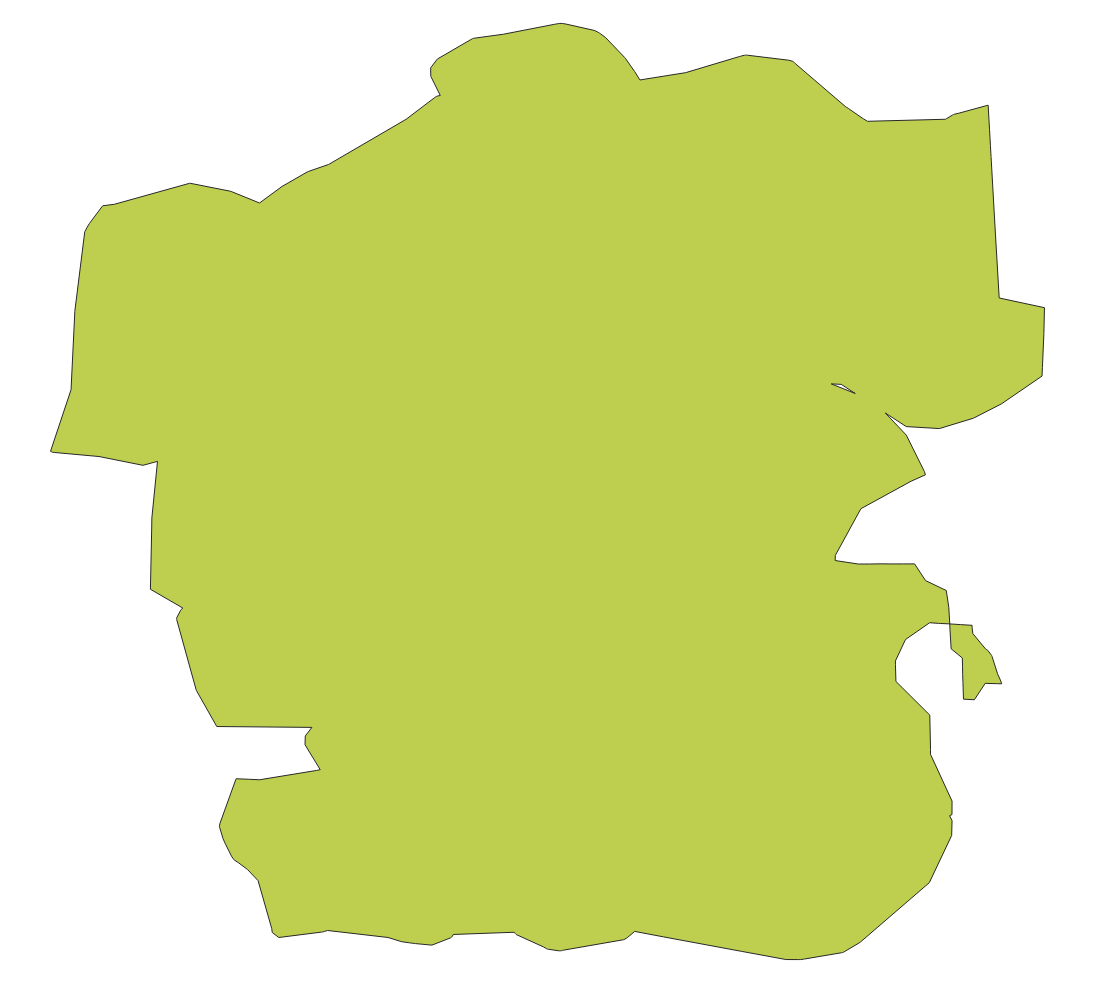# Sizing up and simplifying shapes with fio-planet¶

Here are a few examples related to the Planet Developers Blog deep dive into simplifying areas of interest. The examples use a 25-feature shapefile. You can get it from rmnp.zip or access it in a streaming fashion as shown in the examples below.

## Counting vertices in a feature collection¶

The builtin `vertex_count` function, in conjunction with fio-map's `--raw` option, prints out the number of vertices in each feature. The default for fio-map is to wrap the result of every evaluated expression in a GeoJSON feature; `--raw` disables this. The program jq provides a nice way of summing the sequence of numbers.

``````fio cat zip+https://github.com/planetlabs/fio-planet/files/10045442/rmnp.zip \
| fio map 'vertex_count g' --raw \
28915
``````

Here's what the RMNP wilderness patrol zones features look like in QGIS.## Counting vertices after making a simplified buffer¶

One traditional way of simplifying an area of interest is to buffer and simplify. There's no need to use jq here because fio-reduce prints out a sequence of exactly one feature. The effectiveness of this method depends a bit on the nature of the data, especially the distance between vertices. The total length of the perimeters of all zones is 889 kilometers.

``````fio cat zip+https://github.com/planetlabs/fio-planet/files/10045442/rmnp.zip \
| fio map 'length g' --raw \
889332.0900809917
``````

The mean distance between vertices on the edges of zones is 889332 / 28915, or 30.7 meters. You need to buffer and simplify by this value or more to get a significant reduction in the number of vertices. Choosing 40 as a buffer distance and simplification tolerance results in a shape with 469 vertices. It's a suitable area of interest for Planet APIs that require this number to be less than 500.

``````fio cat zip+https://github.com/planetlabs/fio-planet/files/10045442/rmnp.zip \
| fio reduce 'unary_union c' \
| fio map 'simplify (buffer g 40) 40' \
| fio map 'vertex_count g' --raw
469
``````## Counting vertices after dissolving convex hulls of features¶

Convex hulls are an easy means of simplification. There are no distance parameters to tweak. The `--dump-parts` option of fio-map turns the parts of multi-part features into separate single-part features. This is one of the ways in which fio-map can multiply its inputs, printing out more features than it receives.

``````fio cat zip+https://github.com/planetlabs/fio-planet/files/10045442/rmnp.zip \
| fio map 'convex_hull g' --dump-parts \
| fio reduce 'unary_union c' \
| fio map 'vertex_count g' --raw
157
``````## Counting vertices after dissolving concave hulls of features¶

Convex hulls simplify, but also dilate concave areas of interest. They fill the "bays", so to speak, and this can be undesirable. Concave hulls do a better job at preserving the concave nature of a shape and result in a smaller increase of area.

``````fio cat zip+https://github.com/planetlabs/fio-planet/files/10045442/rmnp.zip \
| fio map 'concave_hull g :ratio 0.4' --dump-parts \
| fio reduce 'unary_union c' \
| fio map 'vertex_count g' --raw
301
``````